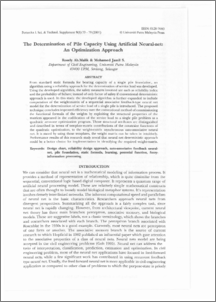# The Determination of Pile Capacity Using Artificial Neural-net: An Optimization Approach

## Citation

Ab. Malik, Rosely and Jamil S., Mohamed (2001) The Determination of Pile Capacity Using Artificial Neural-net: An Optimization Approach. Pertanika Journal of Science & Technology, 9 (1). pp. 73-79. ISSN 0128-7680

## Abstract

From standard static formula for bearing capacity of a single pile foundation, an algorithm using a reliability approach for the determination of service load was developed. Using the developed algorithm, the safety measures involved are such as reliability index and the probability of failure; instead of only factor of safety if conventional deterministic approach is used. In this study, the developed algorithm is further expanded to include computation of the weight-matrix of a sequential associative feedback-type neural net model for the determination of service load of a single pile is introduced. The proposed technique concludes improved efficiency over the conventional method of commissioning the functional formula of the weights by exploiting the structural properties of the matrices appeared in the codification of the service load to a single pile problem as a quadratic zero-one optimization program. Those structural attributes are distinguished and described in terms of template-matrix contributions of the constraint functions of the quadratic optimization, to the weight-matrix asynchronous auto-associative neural net It is stated by using those templates, the weight matrix can be taken in intuitively. Performance results of this research study reveal that neural net deterministic approach could be a better choice for implementation in identifying the required weight-matrix.Preview
PDF
The_Determination_of_Pile_Capacity_Using_Artificial_Neural-net.pdfView Item